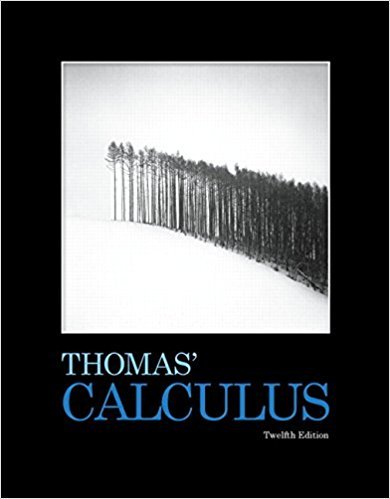×
×

# Solutions for Chapter 12: Vectors and the Geometry of Space## Full solutions for Thomas' Calculus | 12th Edition

ISBN: 9780321587992Solutions for Chapter 12: Vectors and the Geometry of Space

Solutions for Chapter 12
4 5 0 414 Reviews
15
1
##### ISBN: 9780321587992

Since 76 problems in chapter 12: Vectors and the Geometry of Space have been answered, more than 8172 students have viewed full step-by-step solutions from this chapter. This textbook survival guide was created for the textbook: Thomas' Calculus, edition: 12. This expansive textbook survival guide covers the following chapters and their solutions. Thomas' Calculus was written by and is associated to the ISBN: 9780321587992. Chapter 12: Vectors and the Geometry of Space includes 76 full step-by-step solutions.

Key Calculus Terms and definitions covered in this textbook
• Absolute maximum

A value ƒ(c) is an absolute maximum value of ƒ if ƒ(c) ? ƒ(x) for all x in the domain of ƒ.

• Bounded above

A function is bounded above if there is a number B such that ƒ(x) ? B for all x in the domain of ƒ.

• Circular functions

Trigonometric functions when applied to real numbers are circular functions

• Combinatorics

A branch of mathematics related to determining the number of elements of a set or the number of ways objects can be arranged or combined

• Cosecant

The function y = csc x

• Direction vector for a line

A vector in the direction of a line in three-dimensional space

• Higher-degree polynomial function

A polynomial function whose degree is ? 3

• Infinite limit

A special case of a limit that does not exist.

• Interval notation

Notation used to specify intervals, pp. 4, 5.

• Midpoint (on a number line)

For the line segment with endpoints a and b, a + b2

• Multiplication principle of probability

If A and B are independent events, then P(A and B) = P(A) # P(B). If Adepends on B, then P(A and B) = P(A|B) # P(B)

• Normal distribution

A distribution of data shaped like the normal curve.

• Polar coordinate system

A coordinate system whose ordered pair is based on the directed distance from a central point (the pole) and the angle measured from a ray from the pole (the polar axis)

• Power-reducing identity

A trigonometric identity that reduces the power to which the trigonometric functions are raised.

An angle in standard position whose terminal side lies on an axis.

• Quotient identities

tan ?= sin ?cos ?and cot ?= cos ? sin ?

• Rectangular coordinate system

See Cartesian coordinate system.

• Tangent

The function y = tan x

• Trichotomy property

For real numbers a and b, exactly one of the following is true: a < b, a = b , or a > b.

• Variation

See Power function.

×